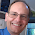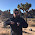## Thursday, October 15, 2015

### When is an intertemporal budget constraint a true budget constraint?

David Glasner cautioned me about the use of an intertemporal budget constraint (since it is based on expectations that could be thwarted) in my emergent representative agent argument (that parallels Gary Becker's argument that even irrational agents can behave rationally). If you have no idea what I am talking about, you should start here. At that link, I said I would address this issue in a future post -- this is that post.

The question is whether we can pretend the intertemporal budget constraint is a true budget constraint analogous to a single period budget constraint for the purpose of constraining the (intertemporal) opportunity set (as Becker puts it). I called the opportunity set the simplex. The issue is that recessions happen and output falls below its expected value (plans are thwarted). That means that expected value isn't a true constraint (actual output is much less) and therefore the most likely state of the economy might not:
• Manifest consumption smoothing
• Saturate the intertemporal budget constraint
• Have downward sloping demand curves
• (Approximately) maximize utility
The crux of the argument showing these hold (if we ignore recessions) with random consumption is that the most likely point of a high-dimensional simplex is on its surface, rather than in the interior. The downward sloping demand curves hold as long as the centroid of the simplex isn't close to any particular axis.

Let's take the opportunity set defined by the intertemporal budget constraint Σ ci ≤ M (see the picture at the top of this post). For d dimensions (time periods) we have

Σ ci ~ M d/(d + 1)

If d >> 1, then we have Σ ci ~ M and the above properties hold: we have emergent rational agents.

What happens if we introduce recessions? Let's take a set of {ci} of size n to be zero: all plans are thwarted in those periods and there is no income ... a 100% recession. Note that for even a bad recession, you're really looking at 20%, not 100%, so this will be a conservative calculation. We can show

Σ ci ~ M (d - n)/(d + n + 1)

However, if d >> n and d >> 1, we still recover Σ ci ~ M. In fact, we still recover all of the properties listed above (if d >> n, then the centroid isn't close to a typical axis and you have downward sloping demand curves almost always). Basically, as long as the economy isn't in a recession most of the time, the intertemporal budget constraint can be treated as an ordinary budget constraint.

Note that the properties hold even if the consumption sets are of different size (i.e. there is economic growth) as long as there is no cj >> ck for most k (in which case Σ ci ~ cj). That case is a lopsided simplex (the opportunity set would be more like a spike along the dimension j).  Can growth make our simplex lopsided? The Euler equation says that successive consumption periods are related by the rate of interest  and we have:

ci ~ β (1 + R) cj

This means that the rate of interest can't be too large. Large here though is a bit extraordinary; we'd need

β c0 (1 + R)^d >> c0 (1 + R)^(d - 1)

or

β (1 + R) >> 1

to make a truly lopsided simplex.

So the final takeaway is that we can treat the intertemporal budget constraint like a true budget constraint in order to demonstrate emergent rationality from random behavior. The caveats are:

• The economy is not in a recession in most time periods
• Consumption isn't concentrated in a few time periods

It is true that when you look at time periods near recessions, things are more interesting. However that is the point of non-ideal information transfer. In the information equilibrium model, information equilibrium is taken to hold most (but only most) of the time.

1.Jason, apologies for the off-topic comment.

I finally made myself work through the expected value of total spending for a randomly-behaving agent subject to a budget constraint spending on k commodities. I worked out the volume of a unit right k-simplex, which is (as you know) the space of feasible points where each point is a k-vector comprising the amount spent on each of the k commodities assuming the sum of the money spent is less than or equal to 1. The result I got is simply 2^(-1/k), which means in one dimension, you spend half your money on average; two dimensions, 71%; and by the time you get to 50 or so dimensions, you're probably spending 99% of your money.

Assuming I did this analysis right, it raises in my mind a question: why do we assume, a priori, that each point within the simplex is equally likely? I know people who basically spend their money on apparently random things until they have none left. This behavior is still nice and random (to me at least), uniformly distributed across the simplex surface furthest from the origin, rather than uniformly distributed across the simplex's full volume as I think you are assuming.

Maybe it makes no difference and the ITM gives you the same result with either consumer model. But I'm still curious.

Thanks,
-Ken

1.Hi Ken,

It's not really off-topic. Treating each point as equally likely is the principle of indifference, and is basically equivalent to several different formulations of the fundamental postulate of statistical mechanics:

https://en.wikipedia.org/wiki/Statistical_mechanics#Fundamental_postulate

The uniform distribution is the maximum entropy distribution assuming you have a maximum value (our budget constraint), however there are other maximum entropy distributions (that depend on your constraint) ... including the partition function, which maximizes entropy given a constraint on the average value. We could say the intertemporal constraint is the average of a large system of agents instead of it being the a strict boundary. That actually might be more realistic because banks can create money through loans, so the budget isn't really a hard wall.

That would probably be the optimal way to deal with this problem and it would still lead to occupying many of the states near the surface (because there are more of them that are indistinguishable), but the distribution of points would look more like a Maxwell-Boltzmann distribution with some tail that exceeds the budget wall.

The primary reason for the uniform distribution (other than the principle of indifference) is that it is easy to work with. I like to think of it as the Einstein model vs the Debye model:

https://en.wikipedia.org/wiki/Einstein_solid

The Einstein model gets the basic physics right (freezing out of phonon degrees of freedom), but the Debye model is more accurate about how the phonons freeze out vs temperature.

Also, the volume of the simplex (the area under the d-1 dimensional budget constraint) is 1/d! so for d >> 1, 1/d! ~ 0. There are no states in the interior!

2.Jason, thanks as always for the detailed reply.

I read through the wikipedia pages you linked to, and I have to admit it mostly brought back memories of not doing very well in statistical mechanics as an MIT undergrad... hopefully I will figure all of this stuff out someday.

> There are no states in the interior!

Yes, understood. It doesn't matter what probability distribution across the state space you use as d grows large, you'll wind up spending most of your money most of the time for any reasonable distribution.

3.Jason, I love how much mileage you've gotten out of that simplex diagram.

Comments are welcome. Please see the Moderation and comment policy.

Also, try to avoid the use of dollar signs as they interfere with my setup of mathjax. I left it set up that way because I think this is funny for an economics blog. You can use € or £ instead.